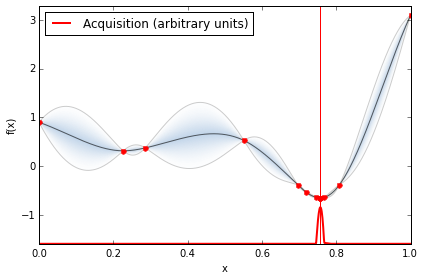GPyOpt is very easy to use and has been developed in a way that can be by for both newbies and expert Bayesian optimization users. It has two main interfaces. You can solve your problems using the Python console of loading config files.

GPyOpt using the Python console

This is an example of how to use GPyOpt in the Python console. The following code defines the problem, runs the optimisation for 15 iterations and visualize the results.

from GPyOpt.methods import BayesianOptimization
import numpy as np

def f(x): return (6*x-2)**2*np.sin(12*x-4)
domain = [{'name': 'var_1', 'type': 'continuous', 'domain': (0,1)}]

myBopt = BayesianOptimization(f=f, domain=domain)
myBopt.run_optimization(max_iter=15)
myBopt.plot_acquisition()GPyOpt from config files

You can also solve your problems via the Linux console. To start, create a directory /myproblem and define your objective function and problem config files in separate files.

The problem definition should be a .py file. This is an example of a file that contains the Branin function.

myfunc.py

def myfunc(x,y):
return (4-2.1*x**2 + x**4/3)*x**2 + x*y + (-4 +4*y**2)*y**2

In a json file, configure the parameters of the optimization. Details of the different options can be found in the reference manual. This is an exmaple of a json file that configures the optimization to solve the above defined problem.

config.json

{
"language"        : "PYTHON",
"main-file"       : "myfunc.py",
"experiment-name" : "simple-example",
"likelihood"      : "gaussian",
"resources": {
"maximum-iterations" :  1,
"max-run-time": "NA"
},
"variables" : {
"y" : {
"type" : "FLOAT",
"size" : 1,
"min"  : -3,
"max"  : 3
},
"x" : {
"type" : "FLOAT",
"size" : 1,
"min"  : -2,
"max"  : 2
}
},
"output":{
"verbosity": true
}
}

Now you just need to run the optimization by doing

\$ gpyopt.py ../myproblem/config.json

Once the the optimisation has finished new files with the details of the optimization process should appear in your folder folder:

• Evaluations.txt: containing the locations and values of the function evaluations.
• Models.txt: containing the parameters of all the models used.